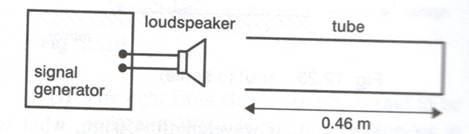CATEGORIES:

Coursework 9 Mechanical waves and stationary waves 2011-12

1) Give an example of each of the following:

i) a longitudinal, progressive, mechanical wave

ii). an electromagnetic, stationary wave

iii) a transverse, mechanical, progressive wave

iv) a transverse mechanical stationary wave

v) a longitudinal mechanical stationary wave

(Give as much information as possible - for example it is not sufficient to give as answer “waves on a spring” since this could be transverse or longitudinal, stationary or traverse). (5 marks)

2) (i) Define the intensity of a wave and show that the intensity of waves emitted from a point source follows an inverse square law. What assumptions do you need to make?

(i) A loudspeaker behaves as a 20 W point source of sound energy.

a) What is the sound intensity at a distance of 4 m from the loudspeaker?

b) At what distance from the loudspeaker does the sound intensity have half the value found in part a)?

c) What is the decibel level at 4 m from the speaker?

(Decibels are referred to a reference level where 0 dB is equivalent to an intensity of 10-12 W m-2 ) (12 marks)

3) Write down the equations of two waves travelling in opposite directions along a stretched string which is parallel to the x-axis. Each wave has the following characteristics:

Amplitude = 0.06 m

Period = 0.15 s

Speed = 120 m s-1

At t = 0 the displacement y = 0

Find the equation of the wave which results from the superposition of these two waves. Calculate the following properties of this resultant wave.

i) The distance between nodes

ii) The distance between antinodes

iii) The maximum and minimum amplitudes

iv) The maximum speed of a particle in the wave (12 marks)

4) A length of elastic is stretched between two fixed points a distance 2.4 m apart. The tension in the elastic is 6.0 N and its mass is 0.12 kg. Find the fundamental frequency of vibration of the elastic and also the frequencies of the second and third harmonics. (6 marks)

5) Many people enjoy singing in the shower because their voice resonates in the cavity of the shower-room.

Consider a shower-room measuring 1.1 m×1.2 m×2.4m. What are the four lowest resonant frequencies of stationary sound waves in such a shower-room?

Assume the velocity of sound in air is 340 m s-1.

(Hint: consider the resonant modes in each of the three perpendicular directions). (8 marks)

6) A pipe has length L = 2.00 m and is open at one end and closed at the other.

a) Sketch diagrams to show the amplitude of vibration of particles as a function of the distance along the pipe for the first two resonant modes. Mark on your diagrams the positions of the nodes and antinodes.

b) Find the wavelength and frequency of these two modes.

(Assume the velocity of sound in air is 340 m s-1).

(8 marks)

7) An aluminium rod 1.20 m long is suspended horizontally by two threads. When one end of the rod is tapped by a hammer, the rod “rings” emitting a note of frequency 2130 Hz.

a) Draw a diagram to show the amplitude of particles in the longitudinal standing wave in the rod at this frequency.

b) Obtain a value for the speed of travelling, longitudinal waves along such a rod.

(6 marks)

8 A student carries out the following experiment to determine the speed of sound in air.

A tube 0.46 m long, closed at one end, is set up with a small loudspeaker facing the open end. The loudspeaker is connected to a signal generator as shown below:The student gradually increases the frequency from a very low value until the column of air in the tube first resonates. This occurs at a frequency of 190 Hz.

a) Draw a diagram to show how the vibrations of the gas molecules in the tube forms a standing wave at this frequency. Mark the positions of any nodes and/or antinodes.

b) Obtain the value for the speed of sound in air according to this experiment

(6 marks)

1. A student at the Christmas party stands 4 m from a loudspeaker where the loudness of the music is 110 dB. How far away from the loudspeaker must the student go to reduce the sound to a pleasant 40 dB? What assumptions do you need to make?

(6 marks)

Date: 2015-01-12; view: 546

 <== previous page | next page ==> Coursework 10 MODERN PHYSICS | Management
doclecture.net - lectures - 2014-2019 year. Copyright infringement or personal data (0.002 sec.)